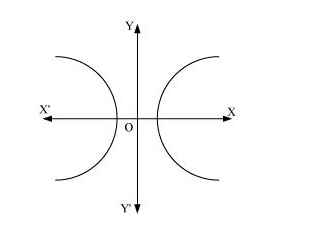# Form the differential equation of the family of hyperbolas having foci on x-axis and centre at origin.

Question:

Form the differential equation of the family of hyperbolas having foci on x-axis and centre at origin.

Solution:

The equation of the family of hyperbolas with the centre at origin and foci along the x-axis is:

$\frac{x^{2}}{a^{2}}-\frac{y^{2}}{b^{2}}=1$                                           ...(1)Differentiating both sides of equation (1) with respect to x, we get:

$\frac{2 x}{a^{2}}-\frac{2 y y^{\prime}}{b^{2}}=0$

$\Rightarrow \frac{x}{a^{2}}-\frac{y y^{\prime}}{b^{2}}=0$                              ...(2)

Again, differentiating both sides with respect to x, we get:

$\frac{1}{a^{2}}-\frac{y^{\prime} \cdot y^{\prime}+y y^{\prime \prime}}{b^{2}}=0$

$\Rightarrow \frac{1}{a^{2}}=\frac{1}{b^{2}}\left(\left(y^{\prime}\right)^{2}+y y^{\prime \prime}\right)$

Substituting the value of $\frac{1}{a^{2}}$ in equation (2), we get:

$\frac{x}{b^{2}}\left(\left(y^{\prime}\right)^{2}+y y^{\prime \prime}\right)-\frac{y y^{\prime}}{b^{2}}=0$

$\Rightarrow x\left(y^{\prime}\right)^{2}+x y y^{\prime \prime}-y y^{\prime}=0$

$\Rightarrow x y y^{\prime \prime}+x\left(y^{\prime}\right)^{2}-y y^{\prime}=0$

This is the required differential equation.